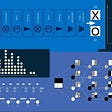# Reinforcement Learning — Beginner’s Approach Chapter -II

Hellow,

In the last chapter, we discussed Reinforcement learning and its applications. A quick recap -

Reinforcement learning is a subset of Machine learning methods in which the agent receives a delayed reward in the next time step to evaluate its previous action. Commonly used in games like Atari and Mario. In Recent Research Reinforcement Learning is embedded with Neural Networks for solving complex tasks.

Again in simple words Reinforcement learning is mapping the situation to actions. The main goal of any reinforcement learning algorithm is to maximize the numerical reward signal. The learner looks for which action will yield the maximum reward instead of which action to take.

I strongly Recommend Readers to hop over Reinforcement Learning — Beginner’s Approach Chapter -I if you are not familiar with basics of Reinforcement Learning .

In this chapter, our main focus will be on breaking Reinforcement Learning Algorithms with Model-free and Model-Based learning.

• Comparison with other ML Algorithm
• Multi Arm Bandit- Framework Introduction
• Markov Decision Process
• Policy optimization or policy-iteration methods
• References

Comparison with Other Machine learning Algorithm

Reinforcement Learning is a subset of machine learning technique that forces an agent to learn in an environment using feedback from an actor’s actions and experiences.

With Supervised Learning-

Reinforcement learning and supervised learning used mapping for input and output In case of Supervised learning correct of actions are required to perform the task but in Reinforcement learning uses rewards and punishment for positive and negative feedback.

Moreover, in supervised learning, we have a supervisor who has complete knowledge of the environment that he will be sharing with the agent to get the task done. But complications arise when we have multiple combinations of subtasks that agents can perform to achieve the objective.

With Unsupervised Learning-

Though both supervised and reinforcement learning use mapping between input and output, unlike supervised learning where feedback provided to the agent is the correct set of actions for performing a task, reinforcement learning uses rewards and punishment as signals for positive and negative behavior. Unsupervised learning analysis takes place in terms of unlabeled data where we find the interconnections between data points and structures them by similarities or differences. However, In Reinforcement learning introduces the best action model to get long term rewards.

With Deep Learning-

RL closely bounded with Deep Learning algorithms as most of RL uses Deep learning Models. Core Methods for Agent to get trained are Neural Networks. Although Neural Nets are best for recognizing complex patterns in images, sounds, and texts.

Multi Arm Bandit- Framework Introduction

As per Wikipedia-

“In probability theory, the multi-armed bandit problem (sometimes called the K or N-armed bandit problem) is a problem in which a fixed limited set of resources must be allocated between competing (alternative) choices in a way that maximizes their expected gain, when each choice’s properties are only partially known at the time of allocation and may become better understood as time passes or by allocating resources to the choice.”

Multi Arm Bandit is a reinforcement learning problem statement in which we have the slot machine consist of n arms or bandits with arms having its own probability distribution of success. If we pull anyone's arm then the result will be the stochastic reward of R =0 for failure and R =+1 for success. The main aim here to pull the arms in such a sequence that it maximizes our total reward.

Certain ways to solve multi-arm bandit problem-

• No exploration: the most naive approach and a bad one.
• Exploration at random
• Exploration smartly with preference to uncertainty

Upper Confidence Bound is one of the famous solutions to the Multi-Arm bandit problem. The algorithm states the principle of optimism with context to uncertainty. Broadly speaking if we are not sure about the selection of arms let’s put our efforts on its exploration more.

For example, we have these four actions which are somehow associated with uncertainties. The agent seems unaware of its action so with the UCB algorithm it will pick up that action which has chances of higher upper bound so that he gets a higher reward for its action moreover he can learn about the actions.

Upper confidence bound has the capability of reducing uncertainty but there is a lag of exploration that comes with time. UCB obtains greater reward on average than other algorithms such as Epsilon-greedy, Optimistic Initial Values, etc.

Markov-Decision Process

According to Wikipedia-

“In mathematics, a Markov decision process is a discrete-time stochastic control process. It provides a mathematical framework for modeling decision making in situations where outcomes are partly random and partly under the control of a decision-maker”

In Simple terms, Markov's Decision process current state has been watched in order to decide the best action by an agent. Before understanding about MDP let’s catch up about several terms -

• Markov Property stated by the following mathematical equation-

State St has the Markov property, if and only if;

P[St+1 | St] = P[St+1 | S1, ….. , St],

Markov Property concludes that if the current state is known and we don’t have any historical information about that state then that state is sufficient enough to provide the same characterization of the future as if we have all the history.

• Markov Process
• Markov chain or process is basically a tuple binding state S and transition function P (S, P). The whole system can be defined by these two components S and P.However we can call it a sequence of random states with Markov Property.
• For example, if you made a Markov chain model of a baby’s behavior, you might include “playing,” “eating”, “sleeping,” and “crying” as states, which together with other behaviors could form a ‘state space’: a list of all possible states. In addition, on top of the state space, a Markov chain tells you the probability of hopping, or “transitioning,” from one state to any other state — -e.g., the chance that a baby currently playing will fall asleep in the next five minutes without crying first.
• Markov chain modeled using the transition matrix to match the transition probabilities. Matrix consist of rows and columns with state-space and cells have their respective probabilities of transitioning from one state to another.

However, MDP is a model for predicting outcomes. It attempts to predict an outcome only when the information is provided by the current state. The Decision-maker has to choose an action available in the current state, resulting in the model moving to the next step and offering the decision-maker a reward.

At each time stamp work of the agent is to perform an action that can change the environment state and agent receiving rewards or penality from the environment. The Agent’s goal is to discover optimal policy i.e. possible set of actions needed to be done in each state that maximizes the total value of rewards received from the environment in response return to its actions. Moreover, MDP is used to put the agent and environment configuration in a formal way.

When a perfect model of the controlled system is available, the MDP problem can be solved using Dynamic Programming (DP) techniques. When the controlled system model is not known, Temporal Difference (TD) techniques can be applied to solve the MDP. Three algorithms for solving the MDP problem are considered: the Q-Learning (QL) and the Fuzzy Q-Learning (FQL) algorithms for finite and infinite state space, respectively.

Policy optimization or Policy-Iteration methods

Before jumping to Policy Iteration let’s touch base the Value Iteration-

Value Iteration is nothing but learning the values for all states and then act according to the gradient. Bellman Update is used to depicts all values learned from value iteration. The Bellman Update is guaranteed to converge to optimal values, under some non-restrictive conditions.

Have been thinking of skipping Maths Behind in this chapter!!!

It has been observed that learning a value might take an infinite amount of time. So Learning a Policy should be the best approach instead of learning value.

Policy Iteration basically looks at the present value incrementally and extracts the policy. It converges faster than Value iteration due to its finite action space. Conceptually, Any changes to actions will happen well before the small rolling-average updates end.

Moreover, there are two types of Policy iteration techniques

• Policy Extraction, which is how you go from a value to a policy-Policy that maximizes above the expected value
• Policy Evaluation. Evaluation is basically done by taking a policy and runs value iteration conditioned on a policy. The samples are forever tied to the policy, but we know we have to run the iterative algorithms for way fewer steps to extract the relevant action information.

Q-value Iteration

Optimal Values can be hard to distill a policy from it. The Q Value Iteration somehow performing value iteration over Q factors. Q -Factors are nothing but simply the state-action value function. Let us take an intuitive example to be S and A be the state and action spaces. Hence applying the state-action value function we take all the state-action (s,a) pairs, for all s in S and a in A, and build a new MDP with transitions between pairs in this extended space.

The reason most instruction starts with Value Iteration is that it slots into the Bellman updates a little more naturally. Q-value Iteration requires the substitution of two of the key MDP value relations together. After doing so, it is one step removed from Q-learning, which we will get to know

Quality-Learning or Q-learning

Let’s put Q -learning/Quality learning in straight and simple- Its an off-policy RL algorithm that helps us to find the best action for a given current state. So What’s its called off-policy algorithm -because it learns from actions that are outside the current policy, like taking random actions, and therefore the policy isn’t needed. Moreover, Q learning also helps us to find the policy that maximized our total reward.

Q-learning more often required preparation of a q-table or matrix that has dimensions of state and action values. The initial values in the matrix table will be zero. After certain update of the matrix table, it becomes a reference table for our agent to select the best action based on the q-value.

Stages of Q-learning

• The agent starts in a state (s) takes an action (a) and receives a reward (r).
• The agent selects action by pointing to Q-table with the maximum value OR random values(epsilon, ε).
• Update q-values.

Q[state, action] = Q[state, action] + lr * (reward + gamma * np.max(Q[new_state, :]) — Q[state, action])

There is a lot more on q-learning but I think its enough to get a head start. Please refer Reference section for more info.

Released by Google Deep-mind A3C is a simpler and robust algorithm that can able to obtain much better scores than any other Reinforcement learning algorithms. Moreover one of the major highlights in A3C is that it has both continuous as well as discrete action spaces.

A3C is quite a vast Reinforce Algorithm to digest hence I will be crunching it to provide a better understanding. Moreover, Please Refer below section for more Readings.

Let’s Break it with Its Algorithm Name-Asynchronous Advantage Actor-Critic (A3C)

• Asynchronous This Algorithm uses multiple agents with each agent to have its own environment copy and network parameters. The communication or interaction between agents took place Asynchronously. It’s similar to real-life scenarios in which each human gains knowledge from the experiences of some other human thus allowing the whole global network to be better.
• Actor-Critic Basically A3C is a combination of both Values -Iteration methods and Policy Gradient methods. It uses the power of both methods in order to bring out a prediction of Value function as well as optimal policy function. Learning Agent, however, is used for updating the value function i.e. Critic to Policy function i.e. Actor. Here Policy function is nothing but the probabilistic distribution of the action space.

• This algorithm is faster and more robust than the Reinforcement Learning Algorithms.
• Can be used for discrete as well as continuous action spaces.
• Due to its architecture of gaining knowledge A3C is efficient enough than other Reinforcement learning techniques.

“Will Continue this Blog Series with Intuitive walkthrough of Deep Reinforcement Learning Algorithms!!!! Stay Tuned”

References

If you like this Post, Please follow me. If you have noticed any mistakes in the way of thinking, formulas, animations, or code, please let me know.

Cheers!

--

--

--

## More from Analytics Vidhya

Analytics Vidhya is a community of Analytics and Data Science professionals. We are building the next-gen data science ecosystem https://www.analyticsvidhya.com

## How & Why Machine Learning Methods Work?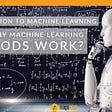## FIRE DETECTION ALGORITHM USING IMAGE PROCESSING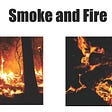## What is Natural Language Processing(NLP) ?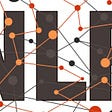## Ensemble Learning case study: Model Interpretability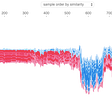## Clustering Tales## Neural text generation## Simplified Machine Learning## Shashwat Tiwari

Senior Applied Data Scientist at dunnhumby || Machine Learning and Deep Learning Ardent ||

## 3 ways to create a Deep Neural Network model with Keras and Tensorflow 2.0## Waste Management using Neural Networks## Artificial Neural Networks